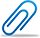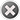Cash-back offer from 1st to 8th February 2023. Get flat 10% cash-back credited to your account for a minimum transaction of \$50. Post Your Question Today!

Question Details Urgent
\$ 5.00

# Math Prob and Stats

• From Mathematics, Probability
• Due on 31 May, 2017 12:00:00
• Asked On 31 May, 2017 10:06:32
• Due date has already passed, but you can still post solutions.
Question posted byDiscussion Board Forum 1/Project 2 Instructions

Standard Deviation and Outliers

For this assignment, you will use the Project 2 Excel Spreadsheet to answer the questions below. In each question, use the spreadsheet to create the graphs as described and then answer the question.

1. A. Create a set of 5 points that are very close together and record the standard deviation. Next, add a sixth point that is far away from the original 5 and record the new standard deviation.

What is the impact of the new point on the standard deviation? Do not just give a numerical value for the change. Explain in sentence form what happened to the standard deviation. (4 points)

B. Create a data set with 8 points in it that has a mean of approximately 10 and a standard deviation of approximately 1. Use the second chart to create a second data set with 8 points that has a mean of approximately 10 and a standard deviation of approximately 4. What did you do differently to create the data set with the larger standard deviation? (4 points)

1. Go back to the spreadsheet and clear the data values from Question 1 from the data column and then put values matching the following data set into the data column for the first graph. (8 points)

50, 50, 50, 50, 50.

Notice that the standard deviation is 0. Explain why the standard deviation for this one is zero. Do not show the calculation. Explain in words why the standard deviation is zero when all of the points are the same. If you don’t know why, try doing the calculation by hand to see what is happening. If that does not make it clear, try doing a little research on standard deviation and see what it is measuring and then look again at the data set for this question.

1. Go back to the spreadsheet one last time and put each of the following three data sets into one of the graphs. Record what the standard deviation is for each data set and answer the questions below.

Data set 1:       0, 0, 0, 100, 100, 100

Data set 2:       0, 20, 40, 60, 80, 100

Data set 3:       0, 40, 45, 55, 60, 100

Note that all three data sets have a median of 50. Notice how spread out the points are in each data set and compare this to the standard deviations for the data sets. Describe the relationship you see between the amount of spread and the size of the standard deviation and explain why this connection exists. Do not give your calculations in your answer—explain in sentence form. (8 points)

For the last 2 questions, use the Project 1 Data Set.

4.      Explain what an outlier is. Then, if there are any outliers in the Project 1 Data Set, what are they? If there are no outliers, say no outliers. (4 points)

5.      Which 4 states have temperatures that look to be the most questionable or the most unrealistic to you? Explain why you selected these 4 states. For each state, give both the name and the temperature. (4 points)

Replies:

\$ 8.00

## [Solved] Discussion Board Forum 1/Project 2

• This solution is not purchased yet.
• Submitted On 31 May, 2017 01:05:431. a) Create a set of 5 points that are very close together and record the standard deviation. Next, add a 6th point that is far away from the original 5 and record the new standard deviation. What is the impact of the new point on the standard deviation? Do not just give a numerical value for the change. Explain what happened to the standard deviation in words. (4 points) Data x 20 0.5 24 0.5 22 0.5 SD 3.16 28 0.5 Mean 24.00 26 0.5 Median 24.00 0.5 0.5 0.5 1 The standard deviation of the 5 values are close together is 3.16. Data x 20 0.5 24 0.5 22 0.5 SD 14.97 28 0.5 Mean 30.00 26 0.5 Median 25.00 60 0.5 0.5 0.5 1 When the value of 60 is added away from the initial set of 5 numbers, the standard deviation of the set of 6 numbers is 14.97. Because the 60 is more distant from the remaining numbers it increases the spread in the series. When the spread in the series increases so does the variability. Consequently the standard deviation increases. b) Create a data set with 8 points in it that has a mean of approximately 10 and a standard deviation of approximately 1. Use the 2nd chart to create a second data set with 8 points that has a mean of approximately 10 and a standard deviation of approximately 4. ...
Buy now to view the complete solutionAttachment
\$ 10.00

## [Solved] Math Prob and Stats A+

• This Solution has been Purchased 2 time
• Average Rating for this solution is A+
• Submitted On 31 May, 2017 02:57:421. a) Create a set of 5 points that are very close...
Buy now to view the complete solutionAttachment
Other Related Questions

No related question exists

#### The benefits of buying study notes from CourseMerits##### Assurance Of Timely Delivery
We value your patience, and to ensure you always receive your homework help within the promised time, our dedicated team of tutors begins their work as soon as the request arrives.##### Best Price In The Market
All the services that are available on our page cost only a nominal amount of money. In fact, the prices are lower than the industry standards. You can always expect value for money from us.